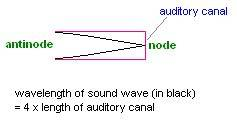Comparison of sound waves in air and underwater

[SOLVED] Comparison of sound waves in air and underwater

Homework Statement

In air, the human ear is most sensitive to sound waves of 3300Hz. The auditory canal is part of the human ear. It is a tube of length 0.025m, i.e. 1/4 the wavelength of sound with frequency 3300Hz.

When under water, the speed of sound is 1500m/s. The human ear becomes most sensitive to sounds with a frequency greater than that of the 3300Hz in air. Explain why this is.

2. Homework Equations and data
Wavelength of sound in air
= 4 x 0.025m
= 0.1m

Speed of sound = wavelength x frequency

The Attempt at a Solution

The auditory canal is a closed tube, the eardrum being the closed end. Thus, the ear is most sensitive to sounds that strike the eardrum at a node:Under water, the ear will still be most sensitive to sounds of wavelength 4 times the length of the auditory canal, i.e. sound of wavelength 0.1m. However, speed of sound under water is greater so the frequency of the sound the ear is most sensitive to must be greater than 3300 Hz.

Frequency x 0.1m = 1500m/s
Frequency = 15000Hz
The ear is thus most sensitive to frequencies of 15000Hz.

------------------------------------
This solution seems to go with the data provided. However, if the sound waves strike the eardrum at a node, shouldn't destructive interference occur? How is the person able to hear anything at all?

Any light into this matter would be really appreciated.Last edited:

Shooting Star
Homework Helper
The ear canal can be treated as open pipe resonating at the most sensitive frequency, and the relationship between length L and f can be roughly estimated by f=v/(2L).

Since v of sound is more in water, f is more, since L is the same.

(EDIT: should be half-open.)

Last edited:
Speed in air
= 0.1m x 3300Hz
= 330m/s

Frequency
= v/(2L)
= (330m/s)/(2x 0.025m)
= 6600Hz

It doesn't match the frequency provided by the question.L is the length of the auditory canal, right? And even if I used wavelength as L, it wouldn't match:

Frequency
= v/(2L)
= (330m/s)/(2x 0.1m)
= 1650Hz

Can we really assume the ear canal to be a open-ended pipe? According to my Barron's SAT book:

Wavelength = 2 x length of open-ended pipe

This contradicts the instructions provided by the question, although if the ear canal were an open-ended pipe the sound waves would strike the eardrum at an antinode (loudest amplitude).

I'm confused.

Last edited:
Shooting Star
Homework Helper
Even if you model the ear canal as a closed tube, then the formula will change to f = v/4L. The point is that f_water/f_air = v_water/v_air => f_water = 15000 Hz, as you had found.

(Your formula from Barron's SAT is identical with what I'd given -- f*lambda=v)

But coming back to your conceptual question, nodes are point of minimal disturbance, not zero disturbance, at least not as far as real sound waves are concerned.

I'm still a little confused. If the ear is a closed tube and we apply the formula as such (wavelength = 4 x length of ear canal), then do all sound waves strike it at a node or do some (with a different wavelength) strike it at antinodes? I suppose if some did strike it at an antinode then the ear would be more sensitive to that?

I'm also wondering about the open tube theory. Can I model the ear as a open tube like you suggested without the formula messing up the frequency like I calculated? What's the difference between modelling it as a closed/open tube?

Shooting Star
Homework Helper
Actually, it's called a half-open tube, I believe. I would think that the ear canal would behave like a half-open tube, seeing that one side is actually open. In a closed tube, nodes are formed at both ends. In a half-open tube, the node is at one end (the eardrum).

You are quite correct, and the ear is sensitive to other resonant frequencies when a node is formed at the eardrum, and an antinode at the other.

Ah, yes... it's a half-open tube. I keep getting my physics vocabulary mixed up. When you said open-ended tube I suppose you meant that the ear canal was actually open at one end but I misunderstood thinking you meant it was open at both ends.

On this vein, do all sound waves strike the eardrum at a node? I suppose that sound of higher resonant frequency and smaller wavelength than the one the ear is most sensitive to must produce overtones, but still strike the eardrum at a node. But what about sound of lower resonant frequency and greater wavelength. Do they strike the eardrum at a position somewhere between a node and an antinode? If so, this sound should be louder than that which strikes the eardrum at a node. Why isn't the ear more sensitive to this?

I'm really sorry if this is off-topic but it seemed interesting and I couldn't find anything helpful on google or my books. Plus my teacher has no idea.

Shooting Star
Homework Helper
The question of node and antinode comes up only when there are standing waves. Standing waves would be formed only for selected frequencies, viz., f = nv/(2L). At these frequencies, the ear would be more sensitive.

But you are quite perceptive in saying that below 3300 Hz, there wouldn't be any standing waves. Perhaps that's why we are not so sensitive to frequencies below that. But there are higher frequencies to which we are sensitive.

Here's something that should delight you:

http://www.kemt.fei.tuke.sk/Predmety/KEMT320_EA/_web/Online_Course_on_Acoustics/hearing.html [Broken]

Last edited by a moderator: